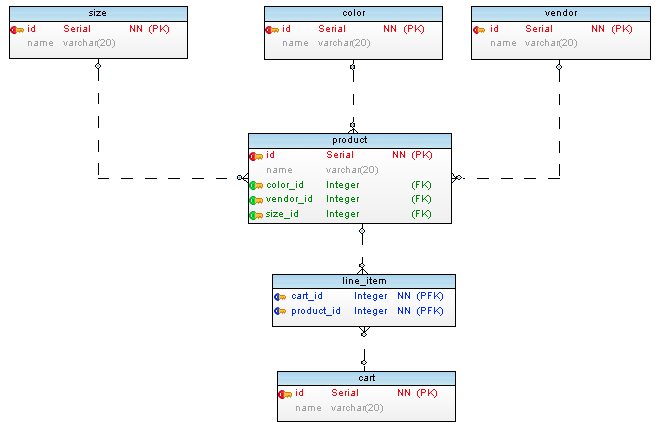## M2ORelation

Kind: global class

### new M2ORelation(args)

Class which represent many to one relationship which resembles `belongsTo` relation in ORMs (Object Relational Mappers). Provides attributes and methods for details of the relationship.

Actually there is no many to one relation in database engine. It is basically one to many relation in reverse direction.

Below is a database schema as an example:

``````size -------------------
id (PK)                |  ---------------------------< line_item >------------ cart
name                   |  |                            product_id (PFK)        id (PK)
|  |                            cart_id    (PFK)        name
^  |
color -------------< product >------------- vendor
id (PK)              id        (PK)         id (PK)
name                 name                   name
color_id  (FK)
size_id   (FK)
vendor_id (FK)

``````

Below is the same schema as image:Some definitions used in descriptions for M2ORelation. Source Table: Table which this relationship belongs to. Target Table: Table that is related to base table.

Param Type Description
args `Object` Attributes of the M2ORelation instance to be created.
args.sourceTable `Table` Source Table which this relation belongs to.
args.targetTable `Table` Target Table which this relation is referring to.
args.constraint `Constraint` Foreign key constraint between source table and target table.
args.namingStrategy `string` Naming strategy to be used.

Example

``````// Example tables have single primary key and examples first relation. So zero index () is used. Use all array elements if necessary.
// line_item >---- product
// (source)        (target)

let relation     = line_item.m2oRelations;            // RELATION:    line_item >---- product
let constraint   = relation.constraint;                  // CONSTRAINT:               ^-- product_has_carts
let sourceTable  = relation.sourceTable;                 // TABLE:       line_item
let targetTable  = relation.targetTable;                 // TABLE:       product
let FKColumn     = relation.constraint.columns;       // COLUMN:      product_id  (from line_item table)
let PKColumn     = relation.targetTable.primaryKeys;  // COLUMN:      id          (from product table)
``````

### m2ORelation.type : `relationType`

Type of relation which is `MANY TO ONE`.

Kind: instance property of `M2ORelation`

### m2ORelation.sourceTable : `Table`

Table which this relation belongs to.

Kind: instance property of `M2ORelation`
Example

``````let relation     = product.M2ORelationRelations;  // RELATION:    line_item >---- product
let sourceTable  = relation.sourceTable;             // TABLE:       line_item
``````

### m2ORelation.targetTable : `Table`

Table which this relation is referred by.

Kind: instance property of `M2ORelation`
Example

``````let relation     = product.M2ORelationRelations;  // RELATION:    line_item >---- product
let targetTable  = relation.targetTable;             // TABLE:       product
``````

### m2ORelation.constraint : `Table`

Foreign key constraint between source table and target table.

Kind: instance property of `M2ORelation`
Example

``````let relation     = product.M2ORelationRelations;  // RELATION:    line_item >---- product
let constraint   = relation.constraint;              // CONSTRAINT:               ^-- product_has_carts
let FKColumn     = relation.constraint.columns;   // COLUMN:      product_id (from line_item table)
``````

### m2ORelation.generateName([strategy]) ⇒ `string`

(! EXPERIMENTAL) Returns name for relation using given strategy. Please see Relation Names for details.

Kind: instance method of `M2ORelation`
Returns: `string` - - Relation name.
See: Relation Names

Param Type Description
[strategy] `string` (simple, complex) Naming strategy to use.